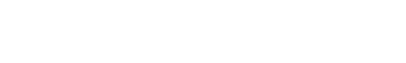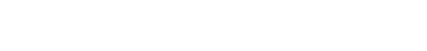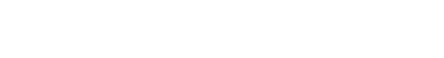# Hydrogen Oxygen Fuel Cell - Electrochemistry

In this video we want to discuss the hydrogen-oxygen fuel cell which is a type of electrochemical cell. We want to learn how to pull the relevant half equations from the Data Booklet without the need to memorise the reactions.

## Determine which species is oxidised and reduced

First we need to determine who is oxidised and reduced. This is simply done by comparing the change in oxidation states for hydrogen and oxygen.

The oxidation state for H increases from 0 in H2 to +1 in H2O. Therefore H2 is oxidised.

The oxidation state for O decreases from 0 in O2 to -2 in H2O. Hence O2 is reduced.The half-equations in the Data Booklet are all written in reduction form.

This means that for the half-equations that we are looking for:

H2 is oxidised hence it is on the right-hand side of the half equation.

O2 is reduced hence it is on the left-hand side of the half equation.

## Acidic Electrolyte H2SO4

For oxidation of H2 we can find 2 half equations where H2 is on the right-hand side:Since the medium is acidic, we need to choose the half equation which contains H+, ie the first one.

Therefore the oxidation of H2 in acidic medium will be as follows:For reduction of O2 we can find 4 half equations where O2 is on the left-hand side:Again we should be choosing the half-equation which contains H+, ie the first one.

Therefore the reduction of O2 in acidic medium will be:The third equation is rejected since O2 will not spontaneously be reduced to H2O2 where oxidation state of oxygen is -1 and unstable.

Finally we can combine both half-equations together and the processes at the anode and cathode in acidic electrolyte are as follows:Ecell is calculated to be +1.23V which is a very easy number to remember. Hence we can use this as a way to verify the half equations we have chosen.

## Alkaline Electrolyte KOH

For oxidation of H2 we can find the same 2 half equations where H2 is on the right-hand side:Since the medium is alkaline, we need to choose the second half equation which contains OH-.

Therefore the oxidation of H2 in alkaline medium will be as follows:For reduction of O2 we can find the same 4 half equations where O2 is on the left-hand side:Again we should be choosing the half-equation which contains OH-, ie the second one.

Therefore the reduction of O2 in alkaline medium will be:The fourth equation is rejected since O2 will not spontaneously be reduced to HO2- where oxidation state of oxygen is -1 and unstable.

Finally we can combine both half-equations together and the processes at the anode and cathode in alkaline electrolyte are as follows:Ecell is calculated to be +1.23V which has the same value in acidic medium.

This is not surprising since the overall redox reaction is the same.

It also reinforces the point that we can easily choose the relevant half equations in acidic and alkaline medium from the Data Booklet instead of blindly memorising them.

Topic: Electrochemistry, Physical Chemistry, A Level Chemistry, Singapore

Back to other previous Physical Chemistry Video Lessons.

## A Level H2 Chemistry Video Lessons

I have a huge collection of short video lessons that targets important H2 Chemistry concepts and common questions.

Join my 3000+ subscribers on my YouTube Channel for new video lessons every week!

Follow me on Instagram for H2 Chemistry videos and (not so funny) memes!

You can also view other A Level H2 Chemistry videos here at my website.

## Need an experienced tutor to make Chemistry simpler for you?

My weekly classes in Singapore are ideal for students who prefer a more structured program.

Build a strong foundation and ace your exams!

Sign up now for a trial lesson at \$50 only (half price promotion)!

Register now and enjoy a promotional locked-in rate of \$360 for a four-week month and \$450 for a five-week month!

Check out the class timing and topics covered for our popular JC1 Classes and JC2 Classes at Bishan, Singapore.

Online lessons are also available! Learn H2 Chemistry anytime, anywhere at 50% of the cost of conventional class tuition.